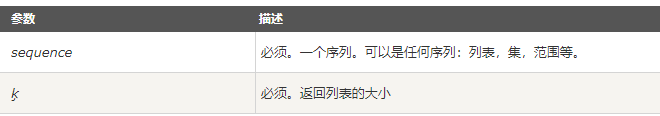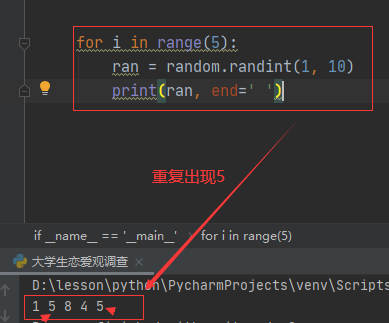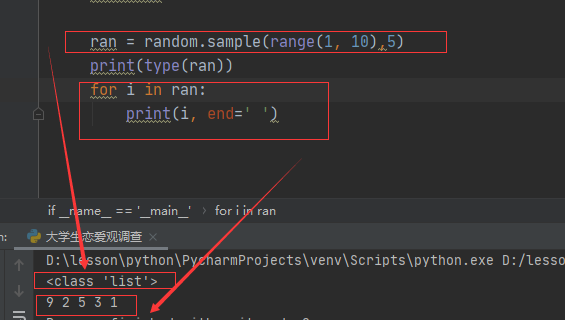• python随机数生成
2021-08-25 09:54:24

# 生成 0 ~ 9 之间的随机数

# 导入 random(随机数) 模块

import random

print(random.randint(0,9))

执行以上代码输出结果为：

4

以上实例我们使用了 random 模块的 randint() 函数来生成随机数，你每次执行后都返回不同的数字（0 到 9），该函数的语法为：

random.randint(a,b)

函数返回数字 N ，N 为 a 到 b 之间的数字（a <= N <= b），包含 a 和 b。

更多相关内容
• 以下的文章主要是以介绍python随机数生成的代码来介绍Python随机数生成在实际操作过程中的具体应用，如果你对其的相关内容感兴趣的话，你就可以点击以下的文章。希望你会对它有所收获。 Python中的random模块用于...
• sample()方法返回一个列表，其中包含从序列中随机选择的指定数量的项目。 random.sample(sequence,k) ... import random #随机数 for i in range(5): ran = random.randint(1, 10) print(r..

sample()方法返回一个列表，其中包含从序列中随机选择的指定数量的项目。

random.sample(sequencek)random.sample(range(1,N), k)
表示从[1,N]的范围内随机生成k个数，结果返回列表

### 如： 随机生成5个(1, 15)范围内的数字：

    import random  #随机数
for i in range(5):
ran = random.randint(1, 10)
print(ran, end=' ')

效果如下:### 如：随机生成5个(1, 10)范围内不重复的数字：

import random

ran = random.sample(range(1, 10),5)
print(type(ran))
for i in ran:
print(i, end=' ')

效果如下:展开全文开发语言 后端
• 1.使用randint生成随机数 import numpy as np ###使用numpy生成随机数:randint A=np.random.randint(0, 10) # [0, 10)之间的随机数 print("A",A) B=np.random.randint(0, 10, 10) ##0-10之间随机数，10个 print("B",...

1.使用randint生成随机数

import numpy as np
###使用numpy生成随机数:randint
A=np.random.randint(0, 10)      # [0, 10)之间的随机数
print("A",A)
B=np.random.randint(0, 10, 10)  ##0-10之间随机数，10个
print("B",B)
C=np.random.randint(0, 2, 10)   ##0-2之间的随机数，10个
print("C",C)
D=np.random.randint(0, 10, size=10)  ##第二种方法
print("D",D)
E=np.random.randint(0, 10, size=(3,5))  ##二维
print("E",E)


输出：

A 0
B [5 3 7 4 9 1 5 4 5 5]
C [0 0 0 0 1 0 1 1 1 0]
D [1 6 5 2 9 6 3 5 1 4]
E [[8 5 6 8 1]
[4 4 5 1 3]
[6 1 4 3 7]]


2.seed随机数种子

##随机数种子的使用，相同的随机数种子能使每次生成的随机数相同，如果不设随机数种子，则值采用系统时间:seed
np.random.seed(10)
F=np.random.randint(0, 10, size=(3, 5))
np.random.seed(10)
G=np.random.randint(0, 10, size=(3, 5))
print("F",F)
print("G",G)


输出：

F [[9 4 0 1 9]
[0 1 8 9 0]
[8 6 4 3 0]]
G [[9 4 0 1 9]
[0 1 8 9 0]
[8 6 4 3 0]]


3.利用random生成随机小数

##不超过1小数随机数：random
H=np.random.random(10)    ##生成10个小数随机数
print("H",H)
I=np.random.random((3,5))   ##小数随机数，二维矩阵
print("I",I)

H [0.00663584 0.3523627  0.00117461 0.61913644 0.87808666 0.66340476
0.94937122 0.01626748 0.78791547 0.41163707]
I [[0.39892079 0.07574883 0.06785014 0.33474267 0.61231963]
[0.20655125 0.38177139 0.12089052 0.36186859 0.40197472]
[0.8976641  0.29853911 0.10580518 0.7516881  0.84469743]]


4.使用normal生成正态分布随机数

##随机生成正态分布数：np.random.normal（均值、标准差、生成数的规模）
X=np.random.normal()  #均值0，标准差1，默认生成1个数
print("X",X)
Y=np.random.normal(10, 12)   ##均值10，标准差，默认生成1个数
print("Y",Y)
Z=np.random.normal(0,10,(3,5))  ##二维正态分布数，均值0，标准差10
print("Z",Z)


输出：

X 0.3891047628993542
Y 7.36819961026948
Z [[ -4.95006355  19.85963067   1.62378056   2.15326265   2.20389965]
[  9.7179342    5.72745771  14.08683078 -13.60423493 -12.43609   ]
[ -9.50769275  14.73418124  18.66561526  16.56514982  15.38531424]]

展开全文• ## python随机数生成

千次阅读 2020-12-17 03:13:53
花开美满Python中的random模块用于生成随机数。下面介绍一下random模块中最常用的几个函数。random.randomrandom.random()用于生成一个0到1的随机符点数: 0 <= n < 1.0random.uniformrandom.uniform的函数原型...花开美满

Python中的random模块用于生成随机数。下面介绍一下random模块中最常用的几个函数。

random.random

random.random()用于生成一个0到1的随机符点数: 0 <= n < 1.0

random.uniform

random.uniform的函数原型为：random.uniform(a, b)，用于生成一个指定范围内的随机符点数，两个参数其中一个是上限，一个是下限。如果a > b，则生成的随机数n: a <= n <= b。如果 a

print random.uniform(10, 20)

print random.uniform(20, 10) \#结果(不同机器上的结果不一样)

18.7356606526

12.5798298022 

random.randint

random.randint()的函数原型为：random.randint(a, b)，用于生成一个指定范围内的整数。其中参数a是下限，参数b是上限，生成的随机数n: a <= n <= b

print random.randint(12, 20) #生成的随机数n: 12 <= n <= 20

print random.randint(20, 20) #结果永远是20

#print random.randint(20, 10) #该语句是错误的。下限必须小于上限。

random.randrange

random.randrange的函数原型为：random.randrange([start],

stop[, step])，从指定范围内，按指定基数递增的集合中 获取一

个随机数。

如：random.randrange(10, 100, 2)，结果相当于从

[10, 12, 14, 16, ... 96, 98]序列中获取一个随机数。

random.randrange(10, 100, 2)在结果上与

random.choice(range(10, 100, 2) 等效。

random.choice

random.choice从序列中获取一个随机元素。其函数原型为：random.choice(sequence)。参数sequence表示一个有序类型。这里要说明 一下：sequence在python不是一种特定的类型，而是泛指一系列的类型。list, tuple, 字符串都属于sequence。有关sequence可以查看python手册数据模型这一章.

print random.choice("学习Python")

print random.choice(["JGood", "is", "a", "handsome", "boy"])

print random.choice(("Tuple", "List", "Dict"))

random.shuffle

random.shuffle的函数原型为：random.shuffle(x[, random])，用于将一个列表中的元素打乱。如:

p = ["Python", "is", "powerful", "simple", "and so on..."] 

random.shuffle(p) 

print p

#---- 结果(不同机器上的结果可能不一样。)

#['powerful', 'simple', 'is', 'Python', 'and so on...']

random.sample

random.sample的函数原型为：random.sample(sequence, k)，从指定序列中随机获取指定长度的片断。sample函数不会修改原有序列。

list = [1, 2, 3, 4, 5, 6, 7, 8, 9, 10]

slice = random.sample(list, 5) #从list中随机获取5个元素，作为一个片断返回

print slice

print list #原有序列并没有改变。

[6, 7, 10, 5, 8]

[1, 2, 3, 4, 5, 6, 7, 8, 9, 10]

展开全文• import random def split(full_list, shuffle=False, ratio=0.2): n_total = len(full_list) offset = int(n_total * ratio) if n_total == 0 or offset < 1: return [], full_list if shuffle: ...
• 随机数生成器，采用Python编写的，对于初学者或者直接利用都可。代码编写逻辑清晰，可以提供很好的利用价值。
• ## python随机数生成

千次阅读 2021-11-26 22:40:02
随机数生成 生成一个随机整数 生成一个3~5之间的整数 import random random.randint(3,5)
• ## python生成随机数

千次阅读 2022-07-12 22:12:52
• 一、概述random模块用于生成伪随机数之所以称之为伪随机数，是因为真正意义上的随机数（或者随机事件）在某次产生过程中是按照实验过程中表现的分布概率随机产生的，其结果是不可预测的，是不可见的。而计算机中的...
• 记录了生成随机数的几种方式以及生成随机列表的几种方法。开发语言
• Python随机数生成 语法： import random #必须导入random模块，才可以调用类似于uniform的函数 利用random模块生成满足几种需求的随机数，如下面几个例子： 例子 如果希望随机数为整数: 方法1：可对其进行类型...
• 一、概述random模块用于生成伪随机数之所以称之为伪随机数，是因为真正意义上的随机数(或者随机事件)在某次产生过程中是按照实验过程中表现的分布概率随机产生的，其结果是不可预测的，是不可见的。而计算机中的随机...
• 随机数生成器pythonWe can use Python random module to generate random numbers. We can use this module to generate random integers, floating-point numbers, or a sequence of random numbers. 我们可以使用...java 机器学习 random 人工智能
• Python is practical language which provides different functions in practical way. Random number are generally used in security related issues but there are other areas too. In this post we will look d...列表 css java random
• 假设你对在Python生成随机数与random模块中最经常使用的几个函数的关系与不懂之处。以下的文章就是对Python生成随机数与random模块中最经常使用的几个函数的关系，希望你会有所收获，以下就是这篇文章的介绍。random...
• 主要介绍了Python使用当前时间、随机数产生一个唯一数字的方法,涉及Python时间与随机数相关操作技巧,需要的朋友可以参考下...